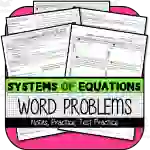Math > Algebra | Grade 8 | Activities

# Systems of Equations: Word Problems NOTES & PRACTICEAttributes
Subject

Algebra

Types

Activities

File

PDF

Editable
No### Systems of Equations: Word Problems NOTES & PRACTICE

A comprehensive teaching resource tool specifically designed for eighth-grade math educators. This resource material meets the common core mathematical standards defined by `CCSS.MATH.CONTENT.8.EE.C.8` and `CCSS.MATH.CONTENT.8.EE.C.8.C`.

#### Focused Content Coverage:

• Analyzing and solving pairs of simultaneous linear equations based in real-world or mathematical scenarios.
• Vital instruction on setting up equations in both Slope-Intercept & Standard Forms framed within mathematical word problems as well as real-world problems.

#### Educational Engagement & Practice Activities:

This product provides practice activities that can be utilized either during class sessions for whole or small group activities, or can be assigned as homework exercises.

#### Included Test Preparation Resources:

Multiple Choice + Constructed Response TEST PRACTICE preparation materials are available for equipping students to tackle assessment challenges.

##### Inclusive Resources Available:
1. Detailed notes on the subject matter
2. Theoretical and applied practice exercises
3. Review materials aimed for test preparation
A perfect blend of practicality and effectiveness, Systems of Equations: Word Problems NOTES & PRACTICE is dedicated to reinforcing algebraic principles in an eighth-grade classroom setting while adhering to standard statutory requirements.

## What's Included

It contains the following resources:

1) Systems of Equations: Word Problems NOTES & PRACTICE

· Understanding how to set up equations in both Slope-Intercept & Standard Forms

· Mathematical Word Problems

· Real World Problems

2) Multiple Choice + Constructed Response TEST PRACTICE

3) Answer Keys to All Parts

## Resource Tags

Systems of Equations Word Problems Algebraic Equations Linear Equations Test Practice

### you may also like...

Check out these other great products